# DL POLY

Jump to: navigation, search

DL_POLY   is a general purpose serial and parallel molecular dynamics simulation package developed at Daresbury Laboratory by W. Smith, T.R. Forester and I.T. Todorov

## Units

DL_POLY employs an interesting set of units which have molecular relevance .:

 physical quantity symbol unit value time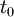$t_0$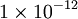$1\times10^{-12}$ seconds (picoseconds) length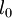$l_0$$1\times10^{-10}$ metres (Angstroms) mass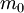$m_0$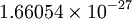$1.66054\times10^{-27}$ kilograms (amu) charge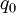$q_0$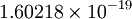$1.60218\times10^{-19}$ Coulombs (electron charge) energy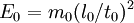$E_0=m_0(l_0/t_0)^2$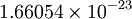$1.66054\times10^{-23}$ Joules = 10 J mol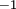$^{-1}$ pressure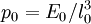$p_0=E_0/l_0^3$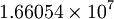$1.66054\times10^{7}$ Pascal = 166.054 bar Planck constant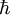$\hbar$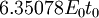$6.35078 E_0 t_0$ Boltzmann constant$k_B$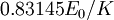$0.83145 E_0/K$

## Force field

The force field used in DL_POLY consists (or can consist) of the following components :

• Chemical bond potentials:
• Angle potentials:
• Dihedral angle potentials:
• Inversion angle potentials:
• Tethering potentials:
• Non-bonded potentials
• Three-body potentials
• Four-body potentials
• Metal potentials
• External fields

## Constraint algorithms

DL POLY can use either the SHAKE or the RATTLE algorithms as well as Q-SHAKE .

## Versions of DL_POLY

The current version of DL_POLY is DL_POLY_4.07

#### Previous versions

DL_POLY_2

DL_POLY_2 was designed for simulations of up to 30,000 atoms and on parallel computers using up to 100 processors.

DL_POLY_3

DL_POLY_3 was designed for simulations of order 100,000 to 1,000,000 atoms running on up to 1000 processors.

• DL_POLY_3 does not handle rigid body molecules.

#### Other versions

DL_MULTI

A DL_POLY package to simulate rigid molecules with multipoles .

## Visualising DL_POLY output

The visualisation program VMD is capable of displaying the HISTORY trajectory file.